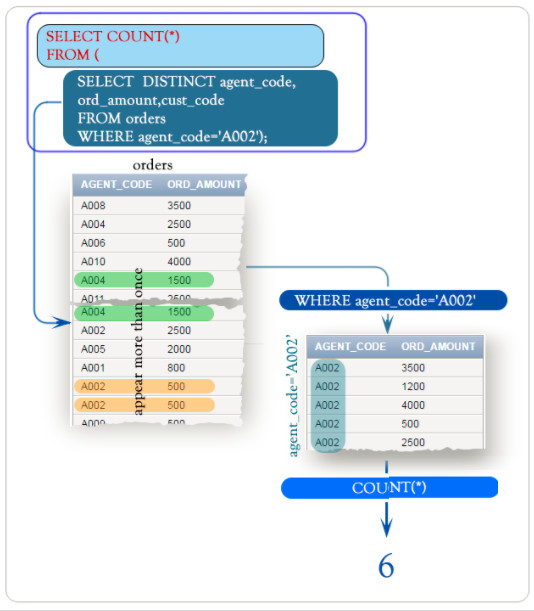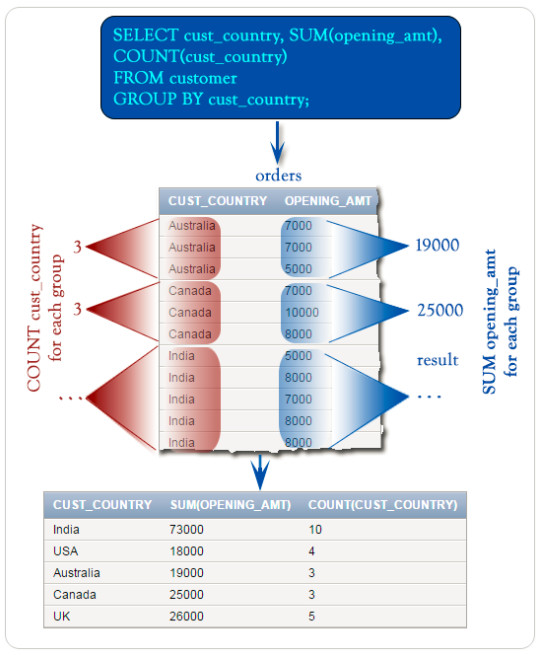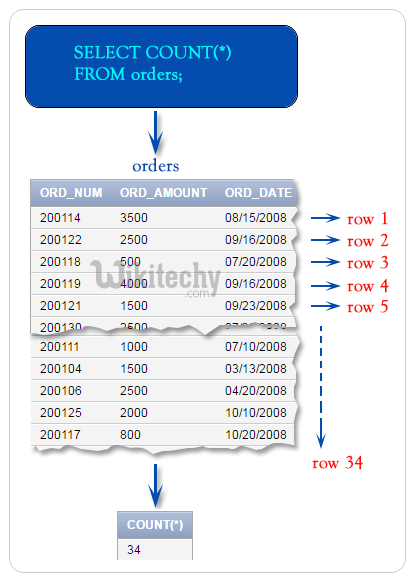# SQL Count | Count functions in SQL - sql - sql tutorial - learn sql

## What is count in SQL• SQL COUNT function is the simplest function and very useful in counting the number of records, which are expected to be returned by a SELECT statement.
• The COUNT () function will return the number of rows that matches a specified criteria.
• The COUNT function in SQL will be used to calculate the number of rows returned from the SQL statement.

## Syntax

• The syntax for the COUNT function is,
• <expression> can be a column name, an arithmetic operation, or a star (*). When we use COUNT(*), we mean "count everything."
• It is also possible to have one or more columns.
• In addition to the COUNT function in the SELECT statement.
• In those cases, these columns need to be part of the GROUP BY clause as well:
• COUNT is often combined with DISTINCT to calculate the number of unique values.
• The syntax for this is as follows:

## Examples

• We use the following table for our examples.

## Table Store_Information

Store_Name Sales Txn_Date
Los Angeles 1500 Jan-05-1999
San Diego 250 Jan-07-1999
Los Angeles 300 Jan-08-1999
Boston 700 Jan-08-1999

## Example 1: Simple COUNT operation

• To find the number of rows in this table

## Result:

• Note:
• We can also use COUNT(*) instead of COUNT(Store_Name).
• In this case the two will generate the same answer because none of the values in the Store_Name field is NULL

## Example 2: COUNT function with a GROUP BY clause• To get the number of records for each store, we type in,

## Result:

Store_Name COUNT(*)
Los Angeles 2
San Diego 1
Boston 1

## Example 3: Use COUNT with DISTINCT

• COUNT and DISTINCT can be used together in a statement to retrieve the number of distinct entries in a table.
• For example, if we want to find out the number of distinct stores in the Store_Information table,

## SQL Count